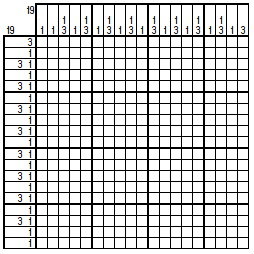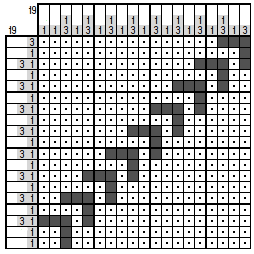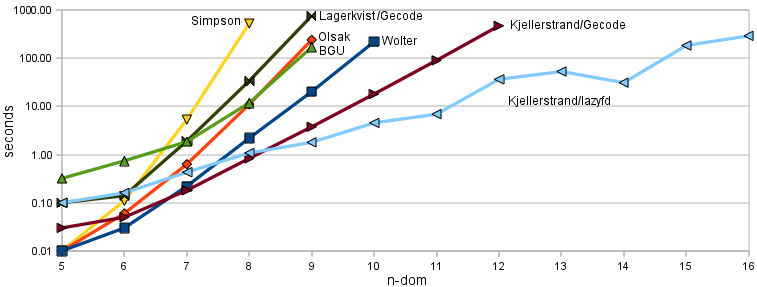# Comparison of Solvers on the n-Dom Puzzles

## Jan Wolter

Designers of paint-by-number puzzle solvers often boast that their solvers use only solution techniques used by humans, so that they can be used to evaluate the appropriateness of a puzzle for solving by humans. This is a nice thought, but it tends to underestimate the creativity of human solvers. Mostly by "human solving techniques" they mean pure line solving, and humans are capable of a great deal more.

"Domino Logic III" is an abstract puzzle design by Josh Greifer in which the solver is forced to do some very unconventional thinking. You can solve this puzzle at the webpbn puzzle site. It is also depicted below.### 1. Manual Solving

How does a human solve this? Here's the solution method Josh had in mind:

Observe that the column clues contain nine 3's. Each of these obviously spans three rows. Note that no two can overlap by more than one row, because any row in which they overlap would have to have more than one black cell, and there are no two consecutive rows that can have more than one black cell.

So, how many rows do we need for all these 3's? We need three rows for the first one, and then two more rows for each additional one, since they can't overlap the other 3's by more than one row. So we need 3+8*2 rows for the nine 3's. That's 19 rows. Well,the puzzle is 19x19, so we only have 19 rows. So there must be part of at least one 3 in every row.

Consider the top row. Which 3's can be in that? Only the one in the right most column, because all the other column clues start with a 1. Thus we know the three in column 19 be in rows 1, 2 and 3. A little simple line solving gets us here:The rest of the puzzle is just a smaller version of the puzzle we started with. You can apply the same logic again, to place the 3 in column 17. We keep doing this, in a sort of domino chain, until we end up with a completed puzzle that can, with a little imagination be viewed as depicting a line of dominos, though the name was inspired by the solution technique, not the image:This kind of logic is entirely different from anything that is ever done by a normal puzzle solving program. It requires quite a holistic approach to the puzzle, instead of focusing on a little bit at a time. There are other solution techniques that are similar in flavor, like the "summing" technique discussed on my advance puzzle solving techniques page.

In my survey of paint-by-number solvers I called this puzzle "9-Dom" because it has nine dominos. You could obviously make similar puzzles of any size. Josh chose this size because it was big enough to make my pbnsolve program (which is used to check all puzzles posted on the site) exceed it's one second time limit and fail.

### 2. Computer Solving

In fact, the n-Dom puzzles cause a lot of solvers problems. Computers are anything but holistic thinkers, so the shortcuts used by humans are unavailable to them. Instead they try to solve this by brute trial-and-error.

Many of them have good enough heuristics that they are able to find the solution in a fraction of a second, but we demand more from our solvers than merely to find a solution. We want them to determine that the solution found is the only possible solution. Many heuristics help to find a path to a solution quickly, but then you still have to examine all other possibilities before you can conclude that the solution is unique. Wilk's solver, for example, solves 9-Dom in a hundredth of a second, but it always halts after finding the first solution, so it isn't really doing the whole job.

Exploring the entire search tree to confirm that there is no other solution can get quite expensive with this puzzle. In many puzzles you can usually find guesses that lead to immediate contradictions, allowing you to mark cells quickly without having to do a lot of searching. On most human designed puzzles with unique solutions pbnsolve can find a contradiction almost all of the time. Not so much in this case, where 7% of the time it fails to do so. That doesn't seem very high, but it's much higher than what is normally seen on anything except puzzles with multiple solutions.

Here are the run times of the various solvers on puzzles 5-Dom through 16-Dom (4-Dom and lower are boring):Note first that the vertical scale is logrithmic. So the nice straight lines of solvers like the Gecode solvers are actually nice exponential explosions. Most of these curves would be going pretty much straight up on a linear scale.

The version of my solver, pbnsolve, used here is 1.07. Version 1.08 solves the Dom puzzles faster, version 1.09 solves it slower.

The most obvious result from this table is that the lazyfd solver is (1) fast, and (2) weird. Yes, it really solves 14-Dom faster than 12-Dom. Some day I'd like to figure out what that thing is up to.

The huge difference between the two Gecode solvers is also interesting. They perform rather similarly on some classes of puzzles, but not here.

Two normally good solvers have unusual problems with the n-Dom puzzles. Both Simpson's solver and the BGU solver show much worse performance on these puzzles than on "normal" puzzles. A partial explanation for why the BGU solver may have this problem appears in my notes on caching line solver solutions.

Given that all of these puzzles are easy to solve for any human who has gotten the key insight, and that increasing size doesn't make them any harder, this isn't exactly a victory for the power of AI.

I'm not sure how much this tells us about the solvers, but the n-Dom puzzles make a nice test set for solvers since they can be scaled arbitrarily large. If your solver has conquered 16-Dom, 100-Dom is still out there waiting for you.